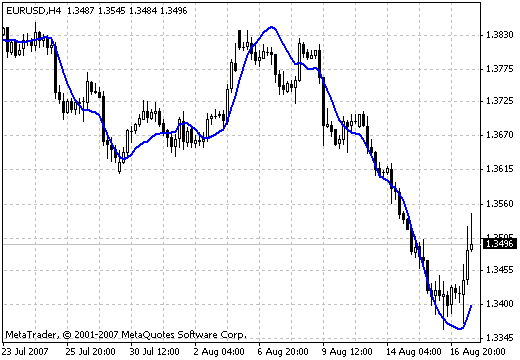# Linear Regression Line – indicator for MetaTrader 4

• A+
category：MT4 INDICATORS

Author: not specified.The mathematical formula expressing the linear regression looks the following way:
у = а + bx, where:
у - close price;
х - position of the current time period in the database;
а - 1/п (Ey - bEх);
b - (nExy - EхEу)/nEх2 - (Ex)2;
п - the number of time periods during the summation;
E - the sum for n periods.

The method of least squares is used for linear regressions in order to fit the line in the data set. The best line is found by minimizing the distance from the data points to this line.